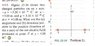# Simple Electric Field Magnitude Question

• jegues

## Homework Statement

See figure attached for problem statement.

## The Attempt at a Solution

I can see that the y-components of each particle will cancel each other out so we are solely looking at how the x-component forces of each particle affect the field at the point P.

These two forces will be added together because they are pointing in the same direction. These forces also happen to have the same magnitude.

So I see the resulting electric field to be as follows,

$$\vec{E_{net}} = - \frac{2kq}{9} \hat{i}$$

I keep getting the magnitude incorrect but I can see that I have the correct angle. (180 degrees)

What am I doing wrong for the magnitude?

EDIT: I found my mistake, I should have done,

$$\vec{E_{net}} = -2 \left( \frac{kqcos\theta}{25} \right) \hat{i}$$

#### Attachments

•CH22-Q11.JPG
41.5 KB · Views: 418
Last edited:
jegues said:
EDIT: I found my mistake, I should have done,

$$\vec{E_{net}} = -2 \left( \frac{kq\cos\theta}{25} \right) \hat{i}$$

How do you define θ ?

You should be able to determine cos(θ) and substitute that into your result.

SammyS said:
How do you define θ ?

You should be able to determine cos(θ) and substitute that into your result.

Where $$\theta = arctan(4/3)$$